# Team:UPO-Sevilla/Model

## MODEL 1: MICROSCOPIC PREDICTION OF BIOFILM GROWTH

### In this simulation, analysis of biofilm formation involves analyzing the bacteria as individual, discrete particles.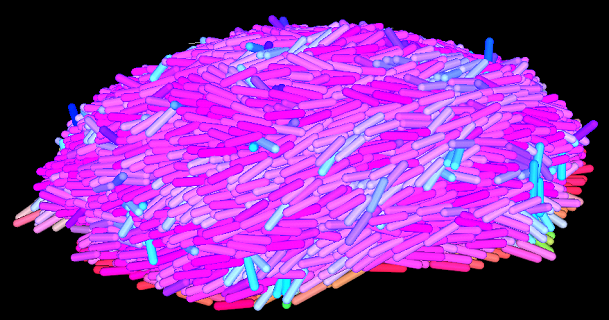Figure 1. Visualization of the software's final output. Starting with one bacteria, a biofilm has been generated.

The prediction starts modelling a 3D liquid space in which one or more bacteria are attached to a solid wall. Notably, these bacteria can reflect in their shape the form of real rod-shaped cells, like Pseudomonas putida. In this way, they are considered cylindrical tubes of length ranging from “L0” to “2L0 + σ”, with semi-spheres of radius “σ/2” at both ends (Figure 2 left).

The software predicts the growth in size of the initial bacteria over time, until the cells reach the critical length (“2L0 + 2σ”). At that point, cell division occurs (Figure 8). This process of growth and division is repeated again and again.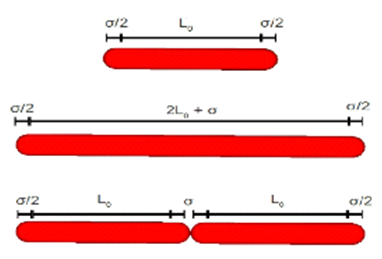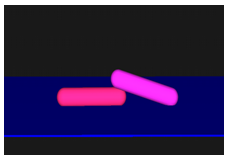Figure 2. Left: Schema of initial cell size (upper part), maximum cell size (medium) and cell division (bottom). Right: Visualization of program's output instants after the first cell division.

### How bacterial consumption of the limiting substrate affects cell and biofilm growth

The velocity in wich, at a given point in time, each cell grows in length is proposed to be: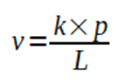Equation 1. Bacterial length growth rate equation.

Where v is the length growth rate of the bacteria in question, k is a proportionality constant, p represents the limiting substrate consumption rate by the cell, and L depicts its length.

Therefore, the length growth rate of a bacterium is inversely proportional to its length. The reason for this is in turn how p is defined: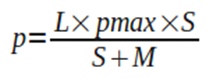Equation 2. Limiting substrate consumption rate equation.

Where pmax corresponds to the maximum length growth rate per bacterial length unit, S is the limiting substrate concentration in the area surrounding the bacterium, and M depicts the limiting substrate concentration at which half of the maximum length growth rate is achieved.

To model that bigger cells would uptake more limiting substrate than smaller cells, equation 2 shows that p is linearly dependent on the length of the bacterium (L). However, the simulation models that bacterial length growth (v) is indepedent from the actual length of the bacterium. As v depends on p and p is dependent on L, it is necessary in turn for v to be inversely proportional to L (equation 1).

Initially, the limiting substrate is evenly distributed in the three dimensional space. However, bacterial consumption creates gradients of the limiting substrate. This causes cells to grow at lower velocities in regions where less limiting substrate is present. At the same time, the software includes standard Fick's diffusion equations to incorporate how these mass gradients spontaneously tend to disappear. That is, how regions (referred to as "sub-areas" in figure 3) where less limiting substrate is present incorporate limiting substrate from adjacent regions by difussion.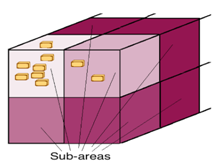Figure 3. Schematic diagram representing how the bacteria consume the limiting substrate in the sub-area they are located. This causes that sub-area to reduce its limiting substrate concentration, and limiting substrate diffusion from neighbouring sub-areas occur. The intensity of the color is proportional to the limiting substrate concentration. Note: This is not a real visualization of the program's output but a diagram.

As a result of equations 1 and 2 and Fick's diffusion, each bacterium in the biofilm grows at its own time-dependent velocity, to reach the critical length and divide.

### What influences bacterial position

The bacteria in the program are attracted to each other and to the solid wall, to reflect the action of adhesins (i.e LapA), which are transmembranal proteins that enable adherance to form a biofilm.

However, it exists simultaneously a certain repulsion force, to emulate in this way the superficial tension of the cells and wall (Figure 4).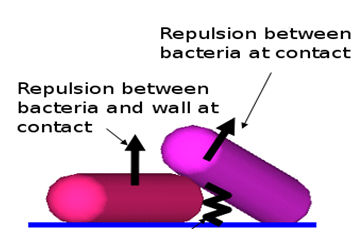Figure 4. Superficial-tension related forces.

At the same time, the position of each cell is also affected by thermal agitation-associated diffusion. Brownian motion equations are included to this end. All these series of factors affect the tridimensional position of each bacterium (r i par), as seen in detail in equations in Figure 5: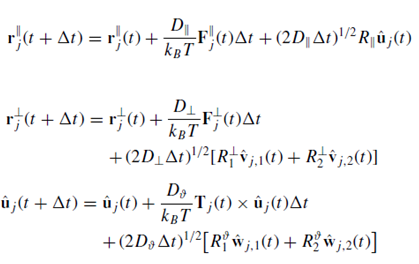Figure 5. Equations determining bacterial position, where r j and r⊥ j indicate the projections of the positions of particle j along ˆ uj and along the directions perpendicular to ˆ uj, respectively; Fj and F⊥ j are the parallel and perpendicular components of the forces, respectively; and R, R⊥ 1 ,R⊥ 2, Rϑ 1 , and Rϑ 2 are independent Gaussian random numbers of variance 1 and zero mean. D, D⊥, and Dϑ are diffusion coefficients. More information at Patti, A. and Cuetos, A. (2012). Brownian dynamics and dynamic Monte Carlo simulations of isotropic and liquid crystal phases of anisotropic colloidal particles: A comparative study. Physical Review E, 86(1).

### Simulation results or output

The simulations take a long time to run as there are a lot of calculations in every time step. For example, it takes around two weeks to create a virtual 17,000 bacteria biofilm, and that making use of a cluster-type super computer. Currently, a new faster version of the software is being developed.

The results can be directly visualized using the opengl application (see above Figure 1), where each bacterium's color depends on its spatial orientation. More interestingly, many biofilm characteristics can be numerically assessed. For example, the average or minimum and maximum growth rates at which bacteria grow in length. Figure 6 is a representation of how these growth rates vary according to the maximum limiting substrate uptake rates (pmax):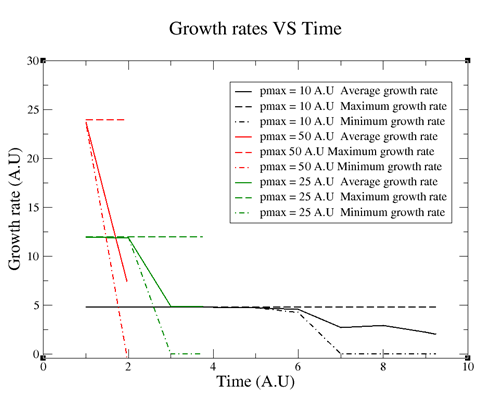Figure 6. Growth rates vs Time according to different pmax values. The final size of the biofilms was 17,000 bacteria. As can be seen, the higher pmax is the less time it takes the biofilm to reach 17,000 bacteria and the higher the growth rate is.

Another parameter which is of importance, as discussed above for biofilm growth, is the limiting substrate concentration in each region of the tridimensional space. Figure 7 depicts the limiting substrate concentration along the surface of the wall on which the biofilm stands.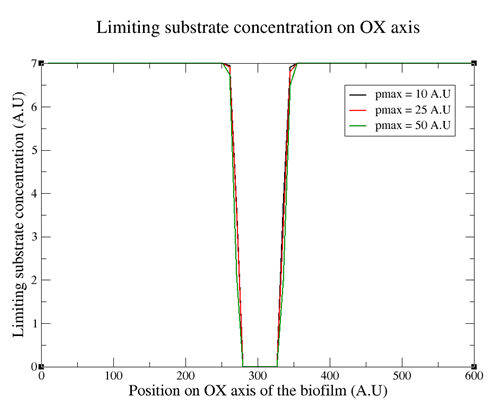Figure 7. Limiting substrate concentration along the OX axis on the surface of the wall where the biofilm is located. The different curves correspond to different pmax values.

Figure 7 shows that the central region on the surface lacks of any limiting substrate concentration. This is due to bacterial consumption. As a result, one conclusion is that the biofilm bacteria that are dividing are only the ones on the outer part of the biofilm and the ones in the center of the biofilm do not grow and divide.

Finally, two curious effects of different maximum limiting substrate uptake rates (pmax) on biofilm shape are shown here. Firstly, how higher pmax reduce the average height of the biofilm (Figure 8).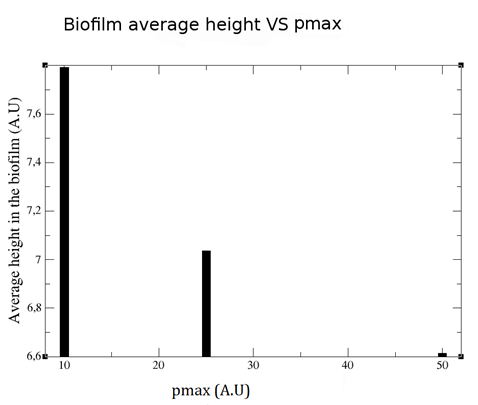Figure 8. Biofim average height vs pmax.

Secondly, how at the same time higher pmax increases the eccentricity of the biofilm. That is, the biofilm shape becomes more linear (less circular) when raising pmax (Figure 9)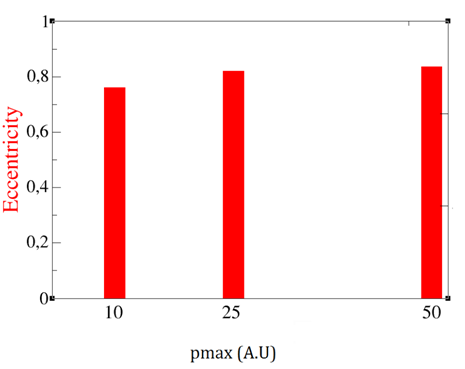Figure 9. Biofilm eccentricity vs pmax.

Therefore, the software has shown some of its potential by analizying the limiting substrate distribution and, interestingly, linking the entire biofilm shape with the uptake rate of limiting substrate by each cell.

## MODEL 2: POPULATION KINETICS OF CULTURES CONTAINING PLANKTONIC AND BIOFILM CELLS

In a bioreactor containing bacteria with the ability to form biofilms, some of the cells will exist in planktonic state and others in biofilm state.

We propose here a model to describe how the planktonic and biofilm populations grow, and how they interact. That is, how a dynamic transition between both states exists. As shown later, this model yet simple has been shown to correspond to experimental results.

In the algorithm, for each time instant in the culture the following calculations take place:

1) Analysis of Biofilm growth rate (Kb) and Planktonic growth rate (Kf):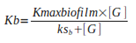Equation 1. Biofilm growth rate (Kb).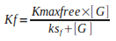Equation 2. Planktonic growth rate (Kf). Where Kmaxbiofilm and Kmaxfree represent the maximum growth rates of the biofilm population and the planktonic population, respectively. [G] depicts the limiting substrate concentration in the bioreactor, ksb and ksf correspond to the limiting substrate concentration at which half of the maximum growth rate is achieved.

Up to now, these equations are equivalent to Monod's growth model. Nevertheless, the next steps make the final results differ from Monod's model.

2) Calculation of the differential of planktonic biomass concentration in the reactor: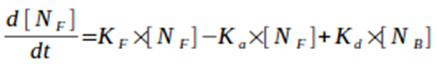Equation 3. Differential of planktonic biomass concentration ([NF]) in the reactor over time (t). Ka is an attachment constant rate of the plantonic cells to the biofilm, Kd is the deatachment constant rate of the biofilm cells and [NB] is biofilm biomass concentration in the reactor.

3) Calculation of the differential of biofilm biomass concentration in the reactor: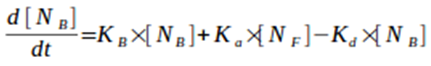Equation 4. Differential of biofilm biomass concentration ([NB]) in the reactor over time. [NF] is planktonic biomass concentration in the reactor.

4) Calculation of the differential of limiting substrate concentration in the reactor, this is inversely proportional to the total biomass generated at each instant :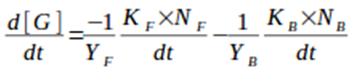Equation 5. Differential of limiting substrate concentration ([G]) in the reactor over time. YF represents the planktonic biomass/limiting substrate yield factor and YB is the biofilm biomass/limiting substrate yield factor.

Notably, the values of the attachment and detachment constant rates (Ka and Kd) vary depending on the limiting substrate concentration in the reactor. Specifically, Ka is higher when above a certain limiting substrate concentration threshold in the reactor and lower when below such threshold. On the other hand, Kd is lower when above the threshold and higher when below. This is done to reflect how bacteria tend to disperse the biofilm when the limiting substrate is at below a certain concentration.

The code and an input example file (named data_values.txt) are available at GitHub UPO Sevilla.

### Comparison between model results and experimental results in the laboratory:

In order to test the validity of the model, we carried out an analysis of Pseudomonas putida behaviour comparing the results obtained with experimental results (Figure 10). As there was no agitation, the limiting substrate in the reactor was dissolved oxygen.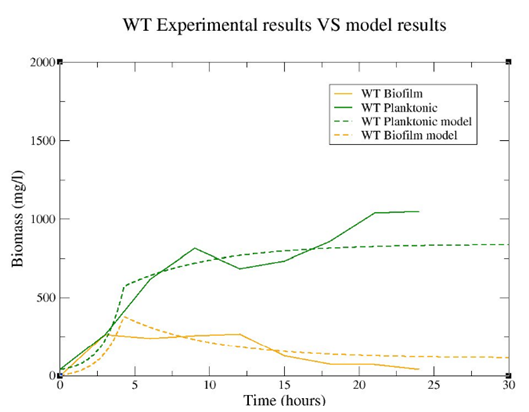Figure 10. Comparison between model results and experimental results in the laboratory. It is important to emphasize that the model curves have been obtained from one only simulation that generated both of them, as the biofilm and planktonic populations are interacting in the model.

Furthermore, an analysis of a biofilm superproducer strain (lapG-) was also carried out. This mutant strain overexpresses the lapA adhesin, which makes it form biofilm in more proportion as bacteria tend to aggregate more. For this purpose, equivalent parameters from the wild type strain was used except that the attachment constant was increased and the detachment constant was decreased (Figure 11). The input parameters file for the mutant strain is also available as "mutant_data" at GitHub UPO Sevilla. Note: In order to run the program, the input parameters file must be first renamed data_values.txt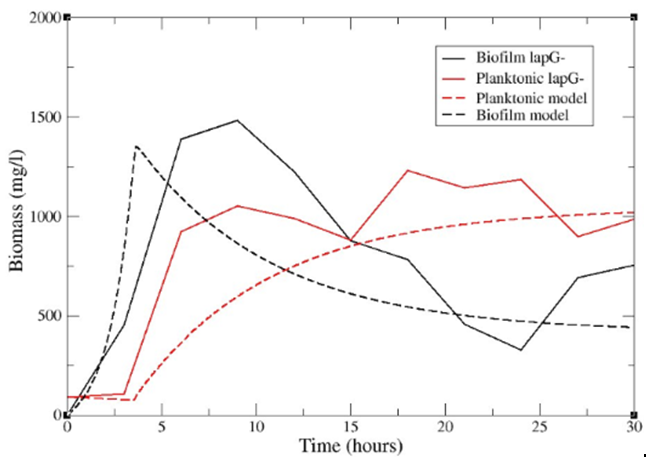Figure 11. Comparison between model and experimental results for lapG- strain.